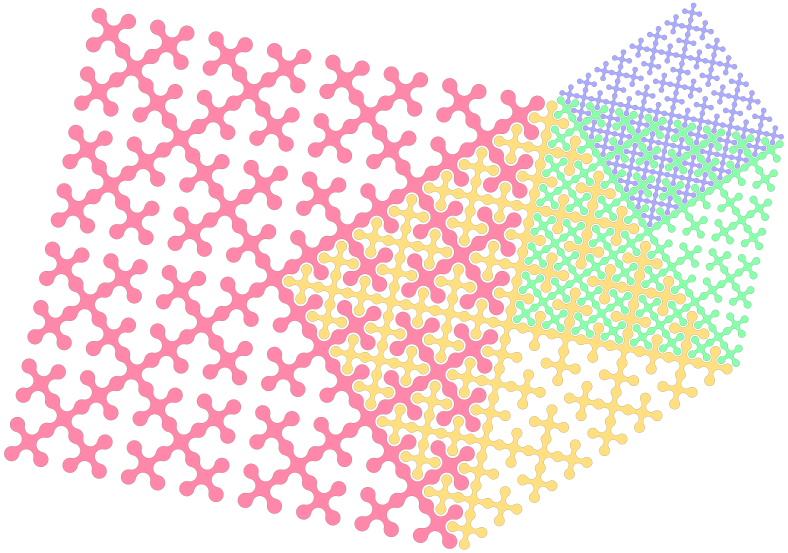# mathr / blog / #

## Calendar 2015 - LungInterlocking space filling curves - in the limit the perimeter between adjacent blocks becomes infinite, like a perfect lung for transferring oxygen from air to blood and carbon dioxide in the other direction. Made with the Haskell Diagrams library:

{-# LANGUAGE TypeFamilies, FlexibleContexts #-}
import Diagrams.Prelude hiding (E)
import Diagrams.Backend.SVG.CmdLine (defaultMain)
import Data.Colour.SRGB (sRGB24)

data H = N | E | S | W

data T = L | R

step L = [L,R,L]
step R = [L,R,R,R,L]

seed = [R,R,R,R]

levels = iterate (concatMap step) seed

angle N =  90 @@ deg
angle E =  0 @@ deg
angle S = -90 @@ deg
angle W =  180 @@ deg

draw d h = (arcD d a a', h')
where
a = angleDir $angle h a' = case d of L -> 90 @@ deg R -> -90 @@ deg h' = heading h d arcD L a b = arc a b arcD R a b = scale (-1)$ arcCW a (rotate b a)

draws = mconcat . (evalState E) . mapM (state . draw)

base
= draws (levels !! level)
# closeTrail
# pathFromTrail
# translate (r2 (-1, 0))
# stroke

left
= base
middle
= base
# scale (sqrt 0.5)
# rotate (45 @@ deg)
# translateX (2 ^ (level + 1))
right
= base
# scale 0.5
# translateX (2 ^ level * 3)
# translateY (2 ^ level)
four
= base
# scale (sqrt 0.125)
# rotate (45 @@ deg)
# translateX (3 * 2 ^ level)
# translateY (2 ^ (level + 1))

lung
= (left # fc r <> middle # fc y <> right # fc g <> four # fc b)
# rotate ((atan 5 :: Double) @@ rad)
# centerXY
# lc black
# lw 0.1
# bg white
# rotate (-90 @@ deg)

y = sRGB24 0xff 0xdf 0x80
r = sRGB24 0xff 0x88 0xaa
b = sRGB24 0xaa 0xaa 0xff
g = sRGB24 0x88 0xff 0xaa

level = 4

main = defaultMain lung